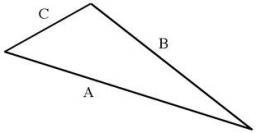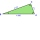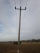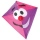# Short cut

Imagine that you are going to the friend. That path has a length 120 meters. Then turn doprava and go another 630 meters and you are at a friend's.

The question is how much the journey will be shorter if you go direct across the field?

Result

x =  108.7 m

#### Solution:

$a=120 \ \text{m} \ \\ b=630 \ \text{m} \ \\ \ \\ c_{0}=a+b=120+630=750 \ \text{m} \ \\ \ \\ c_{1}^2=a^2 + b^2 \ \\ \ \\ c_{1}=\sqrt{ a^2 + b^2 }=\sqrt{ 120^2 + 630^2 } \doteq 30 \ \sqrt{ 457 } \ \text{m} \doteq 641.3267 \ \text{m} \ \\ \ \\ x=c_{0}-c_{1}=750-641.3267 \doteq 108.6733 \doteq 108.7 \ \text{m}$

Try calculation via our triangle calculator.Our examples were largely sent or created by pupils and students themselves. Therefore, we would be pleased if you could send us any errors you found, spelling mistakes, or rephasing the example. Thank you!

Leave us a comment of this math problem and its solution (i.e. if it is still somewhat unclear...):Be the first to comment!Tips to related online calculators
Do you have a linear equation or system of equations and looking for its solution? Or do you have quadratic equation?
Pythagorean theorem is the base for the right triangle calculator.
Do you want to convert length units?

## Next similar math problems:

1. Two parallel chordsIn a circle 70 cm in diameter, two parallel chords are drawn so that the center of the circle lies between the chords. Calculate the distance of these chords if one of them is 42 cm long and the second 56 cm.
2. Diamond area from diagonalsIn the diamond ABCD is AB = 4 dm and the length of the diagonal is 6.4 dm long. What is the area of the diamond?
3. Observation towerFrom the observation tower at a height of 105 m above sea level, the ship is aimed at a depth angle of 1° 49´. How far is the ship from the base of the tower?
4. The right triangleThe right triangle ABC has a leg a = 36 cm and an area S = 540 cm2. Calculate the length of the leg b and the median t2 to side b.
5. Concentric circles and chordIn a circle with a diameter d = 10 cm, a chord with a length of 6 cm is constructed. What radius have the concentric circle while touch this chord?
6. Wall and body diagonalsThe block/cuboid has dimensions a = 4cm, b = 3cm and c = 12cm. Calculates the length of the wall and body diagonals.
7. Medians in RTThe rectangular triangle ABC has a length of 10 cm and 24 cm. Points P, Q, R are the centers of the sides of this triangle. The perimeter of the PQR triangle is:
8. The basesThe bases of the isosceles trapezoid ABCD have lengths of 10 cm and 6 cm. Its arms form an angle α = 50˚ with a longer base. Calculate the circumference and content of the ABCD trapezoid.
9. Telegraph polesThe bases of two adjacent telegraph poles have a height difference of 10.5 m. How long do the wires connect the two poles if the slope is 39° 30´?Calculate the surface of a quadrilateral pyramid, which has a rectangular base with dimensions a = 8 cm, b = 6 cm and height H = 10 cm.
11. Triangular prismCalculate the surface of a regular triangular prism, the edges of the base are 6 cm long and the height of the prism is 15 cm.
12. ProbabilityHow probable is a randomly selected three-digit number divisible by five or seven?
13. FlakesA circle was described on the square, and a semicircle above each side of the square was described. This created 4 "flakes". Which is bigger: the content of the central square or the content of four chips?
14. Annulus from triangleCalculate the content of the area bounded by a circle circumscribed and a circle inscribed by a triangle with sides a = 25mm, b = 29mm, c = 36mm
15. The conicalThe conical candle has a base diameter of 20 cm and a side of 30 cm. How much dm ^ 3 of wax was needed to make it?
16. A kiteChildren have a kite on an 80m long rope, which floats above a place 25m from the place where children stand. How high is the dragon floating above the terrain?
17. Height of the cuboidCuboid with a rectangular base, measuring 3 cm and 4 cm diagonal has a body 13 centimeters long. What is the height of the cuboid?